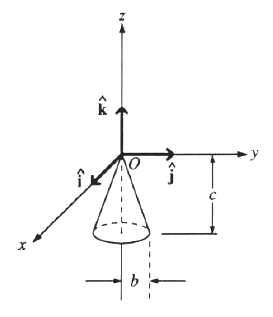GR 8677927796770177 | # Login | Register

GR9277 #8
Problem
 GREPhysics.NET Official Solution Alternate Solutions
\prob{8}A solid cone hangs from a frictionless pivot at the origin O, as shown above. If , , are unit vectors, and a, b, and c are positive constants, which of the following forces applied to the rim of the cone at a point P results in a torque on the cone with a negative component ?

1. , P is (0,b,-c)
2. , P is (0,-b,-c)
3. , P is (-b,0,-c)
4. , P is (b,0,-c)
5. , P is (-b,0,-c)

Mechanics}Torque

The problem wants a negative component for . Recall that whenever and are parallel (or antiparallel). Thus, choices (A), (B), (E) are immediately eliminated. One can work out the cross-product to find that (D) yields a positive , thus (C) must be it.Alternate Solutions
 BerkeleyEric2010-09-14 15:29:40 The z-component of the torque (if you write out the cross product) is . A, B, and E can be immediately thrown out since there is no or component, and then you can just check to see that C gives the write sign.Reply to this commentMall-Saint
2016-10-22 17:08:05
For a torque with a negative z component, the base of cylinder must be rotating clockwise as viewed from above (by the right hand rule). This can be given by a force applied in the y direction to a point on the far side of the base. Hence (C).hooverbm
2012-10-05 08:21:01
For those who are getting confused, for some reason I looked at this problem as if the z-direction were out of the page (force of habit). Clearly z is up/down in the plane of the page.

Torque = r X F and when you determine the direction, point your fingers along the direction of "r" and curl through the smallest of the two angles toward "F". Your thumb is the direction of the torque.

You can go through each option fairly quickly. You soon discover that if F is directed along "y", the only way to get a torque that is in the negative "z" is if the "r" has components into the page (-x direction). Thus C.Setareh
2011-11-08 14:15:47
I really don't understand how you say r and F are parallel in A, B and E...!alimerzairan
2011-10-14 16:31:48
this is just a test of the right-hand rule.

r = thumb

F = index finger

torque = middle finger

we know that the (A), (B), and (E) can't be true, otherwise the cross product will be 0.

And they want the torque to be in the -z direction. So point your middle finger to the -z direction and you find that the r vector must be in the -i direction. This means a negative x-value which is present in (C).jondiced
2010-11-03 14:15:18
Remember that , not ! Oops.BerkeleyEric
2010-09-14 15:29:40
The z-component of the torque (if you write out the cross product) is . A, B, and E can be immediately thrown out since there is no or component, and then you can just check to see that C gives the write sign.
 QuantumCat2014-09-01 12:06:50 That's exactly how I solved this problem. I noted that to get , you have to have a negative crossed with or crossed with negative . Since C & D are the only answer choices with a force not in the direction, and the force in C & D is the in the direction, you must have a negative component, C. Tl;dr: to get you have to have for the given force choices.yosun
2007-06-01 23:07:02
testRebeccaJK42
2007-03-22 11:16:55
Does the R vector point from O to P or P to O?
 yosun2007-06-01 23:06:19 R would point from O to P, of course. Recall the right hand rule, and the two axe vectors ( and ) that spawn from the same origin.LaTeX syntax supported through dollar sign wrappers $, ex.,$\alpha^2_0$produces . type this... to get...$\int_0^\infty\partial\Rightarrow\ddot{x},\dot{x}\sqrt{z}\langle my \rangle\left( abacadabra \right)_{me}\vec{E}\frac{a}{b}\$